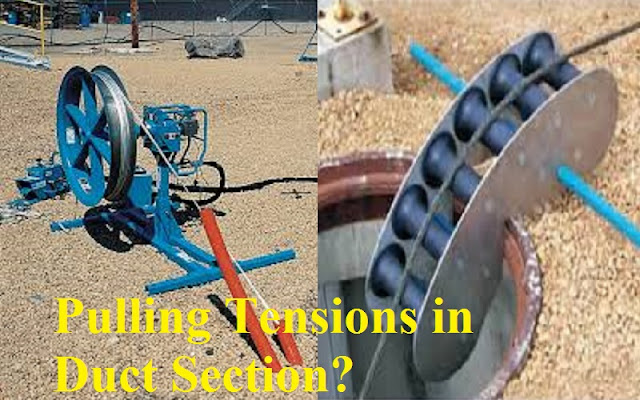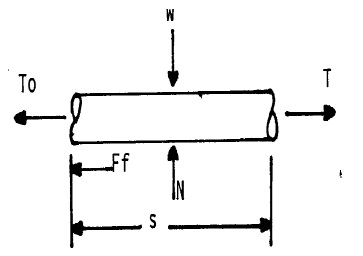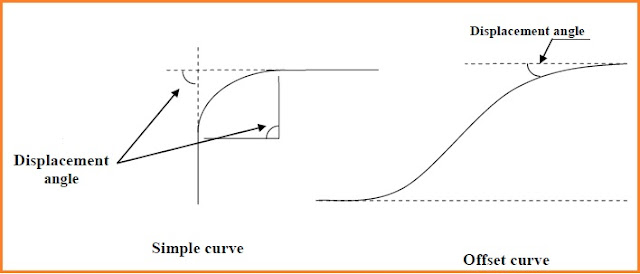How to Calculate Pulling Tensions in Duct Section? - Technopediasite

## Friday, April 12, 2019

How to Calculate Pulling Tensions in Duct Section: I do not say that you will not be aware of pulling tension of fiber optic cable in duct section but today we will try to know about it in detail.This article describes a manual method for calculating the expected pulling tension for underground cable placing.Calculated pulling tensions are used in evaluating whether or not any new underground duct design or existing duct section will cause the expected cable pulling tension to exceed the maximum allowable tension in the cable.

Where the allowable tension would exceed, the duct section must be shortened and / or the bends should be redesigned. It is important that calculations should be made for both the largest and smallest cable that would ever be pulled into the duct section , as often it is a small cable that limits the duct section. The cable pulling tensions ( in both directions ) will be valuable information for the construction forces when choosing the preferred direction of cable pulling.Pulling tension image

## Factors which affect the pulling

The conditions which determine the case or difficulty of pulling cable into duct are very complex. the following are factors which affect the pull :

type and diameter of duct

type and diameter of cable
length of pull
presence and radius of bends in duct
pulling through intermediate manhole
making turns in intermediate manhole
condition of duct ( broken , dirty , flattened ,etc )
whether lubricant is used or not

## Calculating pulling tensions in duct section

The maximum length that a cable can be pulled into a duct is limited by two factors:
The winch line strength or
The strength of the cable

The tension in the winch line fluctuates during the cable pulling in and can attain peak values of twice the normal tension, because of this,duct section length should not be designed to exceed a maximum pulling tension of 18,000 Newton.

The maximum tension that a cable can stand depends on the method by which the winch line is attached. There are two normal ways that the winch line is attached-
(1) By a “ cable grip “ which is slipped over the sheath of the cable , and the sheath takes the stress of the pull.
(2) By a “ pulling eye” which is a steel hook fastened directly to the ends of the conductors , on which all the tension of the pull is applied.

The maximum tension ( tg ) that can be applied with a ” cable grip” can be worked out by using the formula :

Equation (1):
Tg = // ×n× t × ( d-t)
Tg is in Newton // = 3.1416
n = value given by manufacturer (may be 6.9)
T = sheath thickness (in millimeters)
d = sheath overall diameter ( in millimeters)
The maximum tension ( TP ) that can be applied with a “ pulling eye “ can be worked out by using the formula-

Equation (2):
TP = p × 2 × 0.6 × ( value in table 2 )
TP is in Newton
P = number of cable pairs , 2 = tow wires per pair
0.6 = normally only 60% of conductors are terminated
n² = value given by manufacturer (maximum allowable pulling force on a single conductor-8.66, 13.53, 22.87,  43.85).

The two basic equation , when applied in step – by – step fashion, can be used to determine the cable or winch line tension at any point in a duct run composed of any arbitrary succession of straight lengths and bends . the determination for bends should include changes in both horizontal and vertical directions.

The pulling tension necessary to pull a cable through a straight horizontal duct can be calculated using the Equation (3): T = To + fws

Where :T =the pulling forces required at the point of interest. To = the hold back at some reference point. Usually the beginning of the straight section. This is the force created at the reel if the point considered is in the first segment from the reel location. a value of 900 N is considered a reasonable value for this force ( tail load ).

f = coefficient of friction between the cable and the duct
w = mass per unit length of cable (kg / m ) × 9.81 ( to convert to Newton )
s = distance in meters from the reference point to the point of interest.
In case of piece of regular curved duct, the basic Equation

Equation (4)

T = wr × sinh( sinh-1 (To / wr ) + fs/r )

Where T,To,w&f are same as mention in Equation (3)

r = radius of bow curve in meter
h = displacement angle in degrees
s = length of bow curve in meters

Field measurements of pulling tensions suggest the coefficients of friction (consider 0.38).
The calculations assume that the duct will be relatively free of silt and other obstructions at the time the cable is placed.Duct section for FOC route

It can be pull in opposite direction & calculate the tension value and then can take the lower value. Pulling calculation from both sides becomes important. It must be consider the maximum value of pulling tension during the design of ducts sections lengths, not exceed 18000 Newton's.

The following drawing shows the forces affected in the cable during regular tension for it through piece of straight duct :Force affected section

Where: W = mass per unit length of cable (kg / m ) × 9.81 ( to convert to Newton )
N = Normal force in cable created by duct N is equal to W in value & oboist in direction.
Ff = friction force between cable & duct =T × N=T + W × S
T=the force required to displacement the cable through the duct.
TO = the hold back tension.
The force required to displacement the cable must be equal to friction force plus hold back tension.
T = TO + Ff = TO + T × N = TO + T × W × S Equation (3).

## Design of curved sections

For duct build to be useful in perfect way for cable placing in future, it must to be careful during designing of curve sections of duct run because of important of duct bow in pulling tension calculation. The curve section could be in simple curve or offset curve ((S) shape curve). Both shapes of curve sections are shown in below figure.Curve details

Simple curve can be used if it necessary to connect tow pieces of duct of oboist direction to make continue length duct to be useful. Simple curves almost have displacement angles of 90degree or less. Displacement angle almost controlled by the common field status.

Offset curve is used in duct run if there is any prevention in the general direction of ducts, to keep the general direction as it’s with out change. Curved sections of offset curve normally consist of tow bows with equal radiuses. While somebody assuming that the best result ( lowest pulling tension ) depends in increasing radius to maximum range ( 30m usually used ), it’s found that when create an offset curve in certain distance ( S ), the best design can be gotten when bow radius are as smalls as possible, but not lower than 6m, and they connect in share tangent.

Used of small bow radius means make offset curve with little numbers of curved or straight duct sections. This must be executing to reduce the handle tension. Small radius perform to construct offset curves within small area & can be prove best in cases which the available area is limited.

The designer must normally use the larger length that enough to execute offset curve, in which the displacement angle & tension will reduction upon that.

The tension required for pulling of cable through straight section is increase with increasing of section length, so it must be reduce the maximum section length allowed. The value of this reduction is considered as the numbers of curves, displacement angles, and bow radius of each curve & the place of curves in duct section. So it is required to design the curve section to get a cable pulling tension as low as possible.

During the design of curve section ,it must be consider the following factors:

1. In present information 6m bow radius & more is wished.
2. Continue lengths for straight plastic duct of individual standard inner diameter can be only placing as curves shapes when using bow radius of 13m or more and the duct must be fixed in suitable way. In places where the bow radius is between 6m to13m, pieces of 0.5m is used. In case of 6m bow radius or less ready elbow is used.
3. In case of individual inner diameter duct of bow radius less than 25m is used, the duct must be covered with concrete.
4. It must be there of 75mm thickness of the cover in the upper & lower of duct frame. For several ducts of individual inner diameter ducts to be there at the elbows, it must be there 30mm space between tow neighbor ducts vertically & horizontally.

#### 1 comment:

1.